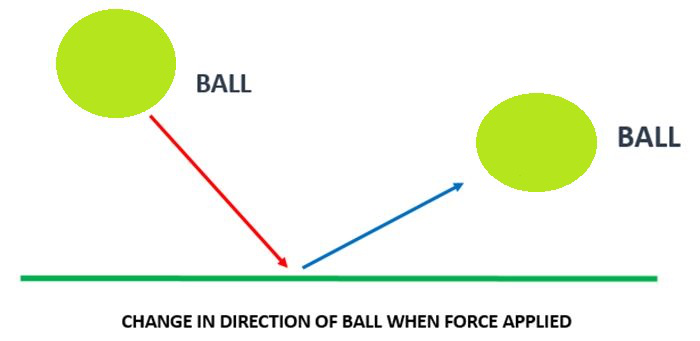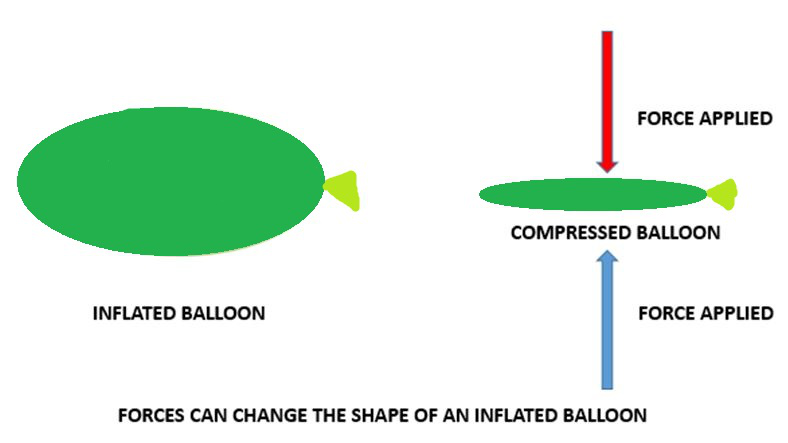Open in App
Not now

# Interaction of Forces

• Last Updated : 20 May, 2021

Before we see into how force can affect the state of motion of a body, let’s take a quick look at the force. As already stated a Force is an interaction between two or more than two bodies that when unopposed to each other cause a change in the state of motion of any object. By the definition of force itself, it very clearly states that force is something that causes a change in the state of motion of the object. Interaction of forces can also be defined as a push or a pull on an object. Any change in the state of motion always corresponds to either a change in the speed of the body and/or a certain change in the direction of motion of the body. A certain change in speed could possibly mean speeding up (acceleration) or in other cases speeding down (deceleration).

For an instance, a ball cannot show motion on its own, nor can a chair or a table. Any non-living objects are themselves stationary or not in any motion unless a force is acting on them. We need to have an engine to generate a force or motion in order to make it move, we need to kick the ball which comes under a form of applied force to let the ball fly into the goal. In many cases, multiple forces can act on the same body or object. Consider that we are kicking a ball and a friend has the exact same idea. What could possibly happen if we both kicked the ball at the same time? The friend or us whoever has a greater interaction of forces build-up will exert a greater force and so will the ball move in that direction.

Remember playing tug-of-war with our friends? In the game of tug-of-war, two teams pull a single rope. The team which is able to pull the rope by a certain amount in spite of the other team pulling it wins the game. Can you guess what exactly is going on there? In that game, two forces are acting in opposite directions with respect to each other. Either of the team is pulling the rope towards the right and another one is trying to pull the rope to the left just as hard and strong as possible. This interaction causes both forces to cancel each other out in the very same way as -3 and +3 cancel each other out. That is only one case. What will happen if forces are acting in the same direction? Do you find it easier to push a car by yourself or with the help of a couple of your friends? It is easier if you push the car together right or not? This is because the force has an interaction that adds up too if applied in the same direction. When we and our friends are pushing in the same direction, all our forces combined are acting on the car hence making it easier to push it together.

To summarize this, if the forces are acting in opposite directions they will cancel each other out and if they will act in the same direction, they will add up. A force changes the motion of an object, i.e. a force can make a stationary body move; it can make a moving object stop; it can increase or decrease the speed of the moving body.

### Factors of Interaction of Forces

Forces when applied in the same direction:

The interaction of forces that act on an object in the same direction always gets added to one another. If the two forces are acting on a body in the same direction then the net force is equal to the sum of the two forces on it. In this case, it always results in a very stronger force than either of the individual forces alone applied.

Example: Two people pushing a heavy car or any heavy box in the same direction. Whenever any heavy load is transported from one place to another many people apply forces in the same direction to move the box.

Forces in the opposite direction:

If the interaction of two forces is acting in opposite directions on a body, the net force acting on it is always the difference between the two forces.

Examples: Consider two workers pushing a heavy load in the opposite direction. The net force on an object is zero if the two forces acting on it in opposite directions are equal in magnitude and if any unequal forces are applied then the box moves in a direction in where a greater force is applied.

People playing in a tug of war pull the rope on opposite sides and whichever team pulls it with a greater force wins.

Magnitude and Direction of a Force:

The interaction of forces could be higher or smaller than another depending upon the strength of magnitude of it. To completely define the action of interaction of force, we have to also specify the direction in which it acts on the body. If the direction or the magnitude of the force changes, its effect also changes accordingly.

For instance, imagine two girls playing the game of circling by holding hands of each other. If there had been no such interaction between them, they could not have been able to move making rounds. The direction and magnitude of forces should be equal and opposite in these case so that each one of them can move with the same speed and enjoy the fullest.

Possible Effects of Interaction of Forces:

An interaction of Force between objects can change the State of Motion of the object:A certain change in either the speed of an object, or its direction of motion, or both, is described as a change in its state of motion.

Example: A fielder catches a cricket ball coming to him with great speed and brings it to rest. Ram hits the ball and changes its direction of motion.

An interaction of Force can Change the Shape of a body:

The shape of a body can be changed using force. We use it to obtain the required shape of the body or object in some cases.

Example: Upon pressing any inflated balloon with two hands compresses or changes the shape of the balloon. While making the dough we change the shape of the dough as we need.

Hence, we can conclude that force can only act on any object, substance, or matter if there exists an interaction between the two bodies. If there is no such interaction between the body and the applied force then the body will not experience any force. As in case someone cannot move a box without touching it. We cannot move a car by simply not touching it. We cannot win the game of tug of war by not touching the rope. All these examples prove that there needs to be an interaction for the forces to act until and unless it is not the case of charged material or bodies in which non-contact interactions exist.### Sample Problems

Question 1: Calculate the force acting on an object when two persons, person A tries to push it by touching it with a force of 60N and from the other person B an opposite force is being applied to stop the object from moving of magnitude 69 N. Calculate the resultant force and the direction to which the object moves.

Solution:

As we know the object will move towards the direction of the force applied by person B as he exerted a greater force.

The resultant force = 69N – 60N = 9N in the direction applied by B.

Hence, the resultant force on the object is 9N.

Question 2: State two examples where we can say interaction between the bodies generated force.

Two examples where we can say interaction between the bodies generated force are:

1. When we try to push a block, we cannot do it without touching it.
2. When we want to move or lift a book we cannot do it without touching it.

Question 3: In a game of cricket a batsman hits the ball with a force of 80N which was coming with a force of 30N. Will the batsman be able to hit the ball at a larger distance? Calculate the net force on the ball.

Yes the batsman will be able to hit the ball as he applied a greater force. The resultant force on the ball = 80N – 30N = 50N. Which will go in the opposite direction from where it was coming.

Question 4: What are interaction force pairs?

In every interaction, there exists a pair of forces acting on the two interacting objects. The direction of the force applied on the first object is opposite to the direction of the force applied on the second object. These forces always come in pairs – equal and opposite action-reaction force pairs.

Question 5: A man was struck with his car in a hole. He tried to push the car alone, but he did not succeed. It was imagined that he applied a force of 40 N to push the car. He called for help. Two people came to help him. One which can apply a force of 70N and another which can apply a force of 10N. From which person should he take help and why? Imagine the reaction force exerted by the car to be 100N.

The man should take the help of person who can apply a force of 70N. As then only the resultant force will be able to overcome the reaction force exerted by the car and the man will be able to move his car.

Given force exerted by the man = 40N

Force exerted by first person = 70N

Force exerted by second person =10N

The resultant force = 40N + 70N = 110N, which is greater than 100N hence it will overcome it.

The resultant force with the other person = 40N + 10N = 50N, which is lesser than 100 hence, it will not overcome it.

My Personal Notes arrow_drop_up